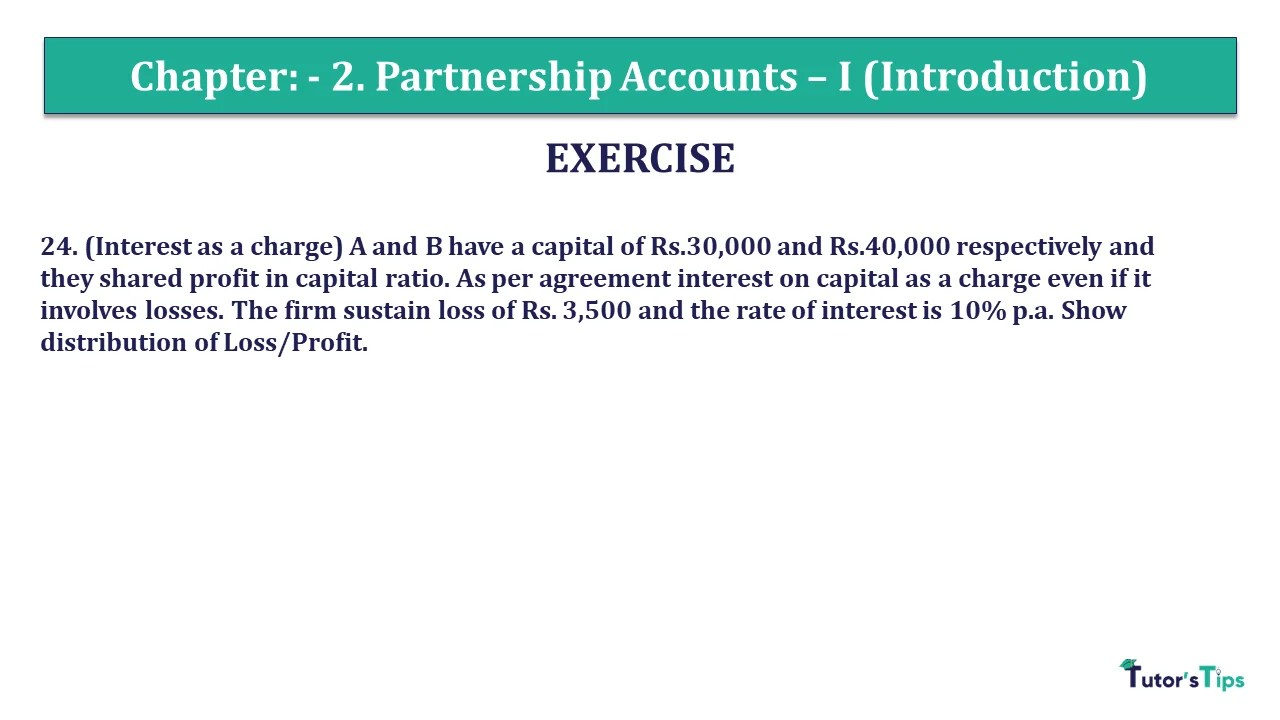# Question 24 Chapter 2 of +2 Part-1 – USHA Publication 12 Class Part – 1Q-24 - CH-2 - Usha +2 Book 2018 - Solution

Question 24 Chapter 2 of +2-Part-1

24. (Interest as a charge) A and B have a capital of Rs.30,000 and Rs.40,000 respectively and they shared profit in capital ratio. As per agreement interest on capital as a charge even if it involves losses. The firm sustain a loss of Rs. 3,500 and the rate of interest is 10% p.a. Show distribution of Loss/Profit.

### The solution of Question 24 Chapter 2 of +2 Part-1: –

 Calculation of net profit Particulars B Loss of firm 3,500 Less: Interest on Capital @ 10% – A (3,000) Interest on Capital @ 10% – B (4,000) Net Loss (10,500)

 Calculation of distribution of profit Particulars A B Net Profit of Rs.10,500 being distributed in 2:1 ratio (4500) (6,000)